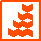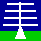HOME

ANNOUNCEMENTS

CONTACTS

DATA

INSTRUMENTS

MISCELLANEOUS

PLOTS

PUBLICATIONS

SCIENCE

WIND-PROFILER
PRINCIPLES

THE NERC MST RADAR FACILITY AT ABERYSTWYTHWARNING: Version-0 MST radar data products are DEPRECATED
Users are encouraged to make use of Version-2 radial files.

File contents
These files contain unaveraged radial (i.e. along-beam) profiles of the radar return parameters (signal-to-noise ratio, signal power, Doppler shift and signal width) for different beam pointing directions.

File naming convention:
rwYYMMDD.tgzYYis a 2-digit year [00 - 99] MM is a 2-digit month [01 - 12] DD is a 2-digit day [01 - 31] tgz indicates that this is a tar file which has been compressed with the GNU gzip algorithm

Click here for the background to the file naming convention, and here to find out how to deal with compressed files. The tar file contains the order of 50 files per day. These individual files have the following naming convention:

rwYYMMDD_hhmm.ddhhis a 2-digit hour [00 - 23] mm is a 2-digit minute [00 - 59] dd is the integer number of minutes covered by the file. The number of digits used is variable and is the minimum number required for the particular file duration.

Archiving convention: YYYY

File availability
1989 to the present.

File format
The first six lines of the individual file rw010903_2142.22 are shown as follows by way of example:

```
4  1  0.80  1  1  1  1    4.0
5  1 11  8 3  320  512  128  1 2  1323
6 101  9  3 21 42 38  18 147   0   0 1
7  18   0.039  0.381   62.4   37.6
7  19   0.024  0.381   66.7   41.9
7  20   0.037  0.381   72.5   47.7
```

The first (integer) number in each line indicates the line type which determines its subsequent contents. Line type 4 occurs only once, at the very beginning of the file, and can be ignored; line type 0 indicates the end of file. Line types 5 and 6 contain header information describing the observational parameters used for each "dwell" (i.e. observations made in a particular beam direction at a given time) and line type 7 contains data at given "range gates" for that dwell. The file therefore contains a line sequence of the form:

4 5 6 7 7 . . 7 7 5 6 7 7 . . 7 7 5 6 7 7 . . 7 7 5 6 7 7 . . . . 7 7 0

The contents of line types 5, 6 and 7 are described in greater detail below. The parameter names shown in red do not actually appear in the files and the inter-parameter spacings (for actual file contents shown in green) have been altered for the sake of clarity

Line type: 5
```  5     1    11  8     3      320  512  128   1    2     1323
LTYP  DWL  BM  PLEN  PCODE  IPP  NCI  LDFT  NII  RXBW  RUN
```
LTYP - Line type
DWL - Dwell number; DWL == 1 corresponds to the beginning of a new cycle of observation
BM - Beam number (in the range 0 - 16) which determines the beam pointing direction
PLEN - Radar pulse length (us)
PCODE - Pulse coding option (0 - no coding; 1 - 8 us sub-pulse coding; 2 - 4 us; 3 - 2 us; 4 - 1 us)
IPP - Inter-pulse period (us)
NCI - Number of coherent integrations
LDFT - Length of discrete Fourier transform (64, 128, 256 or 512)
NII - Number of incoherent integrations

The range resolution of the radar is determined by the receiver bandwidth (RXBW) which, in practice, is matched to the pulse length, or to the sub-pulse length if pulse coding is used:

1 us - 150 m
2 us - 300 m
4 us - 600 m
8 us - 1200 m

Line type: 6
```  6     101  9    3    21  42   38   18   147  0    0    1
LTYP  YR   MTH  DAY  HR  MIN  SEC  RG1  RG2  RG3  RG4  RGI
```
LTYP - Line type
YR - Year of observation; for 1999 YR == 99, for 2000 YR == 100
MTH - Month of observation (0-12)
DAY - Day of observation (1-31)
HR - Hour of observation (0-23)
MIN - Minute of observation (0-59)
SEC - Second of observation (0-59)
RG1 - Bottom range gate of lower altitude region
RG2 - Top range gate of lower altitude region
RG3 - Bottom range gate of upper altitude region; RG3 == 0 for ST-mode observations
RG4 - Top range gate of upper altitude region; RG4 == 0 for ST mode observations
RGI - Range gate interval in multiples of 150 m, i.e. RGI == 1implies samples at 150 m intervals
Line type: 7
```  7     18  0.039  0.381  62.4  37.6
LTYP  RG  DPSH   SPWD   P     S/N
```
RG - Range gate number (between RG1 and RG2, or between RG3 and RG4, inclusive) corresponding to altitudes above mean sea level (km) given by: z = (RG - RG0) × dz

RG0 = 5.2 if (PLEN == 1)
RG0 = 5.7 if (PLEN > 1) & (RXBW == 1)
RG0 = 6.7 if (PLEN > 1) & (RXBW == 2)
RG0 = 8.7 if (PLEN > 1) & (RXBW == 4)
RG0 = 12.7 if (PLEN > 1) & (RXBW == 8)

dz = 0.15 × sin(0°) = 0.1500 - if (BM == 0)
dz = 0.15 × sin(4.2°) = 0.1496 - if (BM == 1) or (BM == 3) or (BM == 5) or (BM == 7)
dz = 0.15 × sin(6.0°) = 0.1492 - if (BM == 9) or (BM == 11) or (BM == 13) or (BM == 15)
dz = 0.15 × sin(8.5°) = 0.1484 - if (BM == 2) or (BM == 4) or (BM == 6) or (BM == 8)
dz = 0.15 × sin(12.0°) = 0.1467 - if (BM == 10) or (BM == 12) or (BM == 14) or (BM == 16)

DPSH - Doppler shift (Hz). These values must be multiplied by (-λ/2), where λ is the radar wavelength (6.41 m), in order to convert them to radial velocities, vR, in (m s-1) for which +ve values imply motion away from the radar, i.e.
vR = -3.20 × DPSH

SPWD - Spectral width (Hz). The definition used to calculate these values (the width of the signal portion of the spectrum containing 80% of the signal power) is non-standard and so the values should be divided by a factor of 2.56 to convert them to the half-width that contains 68.26% of the signal power, i.e. the standard deviation for a Gaussian shaped signal. Moreover, a factor of (λ/2) must be applied to convert these values into velocity units of (m s-1), i.e:
σSW = 1.25 × SPWD

P - Signal power (dB), where P(dB) = 10log10[P(linear)]

S/N - Signal-to-noise ratio (dB); signals for which S/N < 4 dB are too weak for the values of DPSH and SPWD to be relied upon and so these values should be blanked.